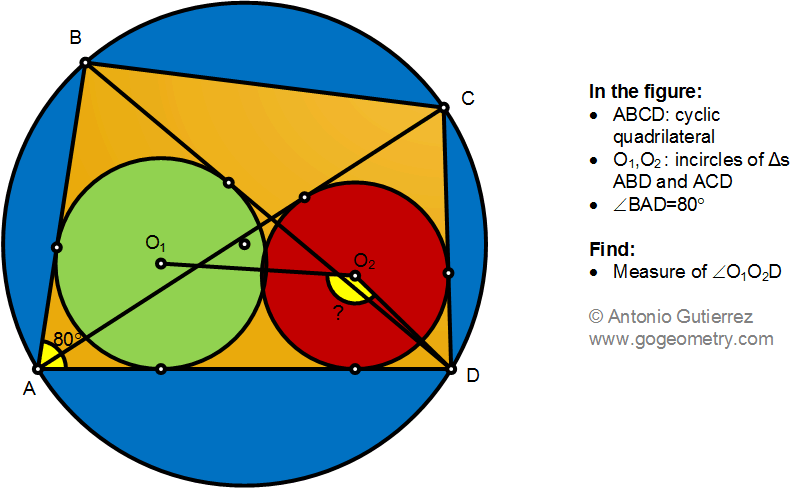# Geometry Problem 1491: Cyclic Quadrilateral, Diagonal, Incircle, Angle, Measurement

The figure shows a cyclic quadrilateral ABCD with the incircles O1 and O2 of the triangles ABC and ACD. If the measure of angle BAD is 80 degrees find the measure of angle O1, O2D.#### Incircle

Incircle is a circle within a triangle, that is tangent to each side.